# AI学习笔记——Tensorflow中的Optimizer(优化器)

+关注继续查看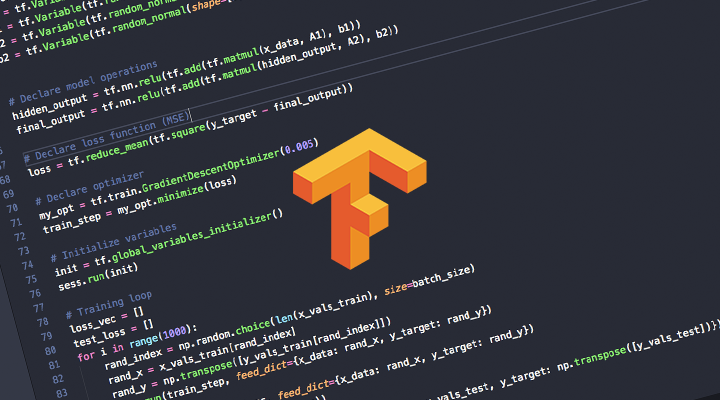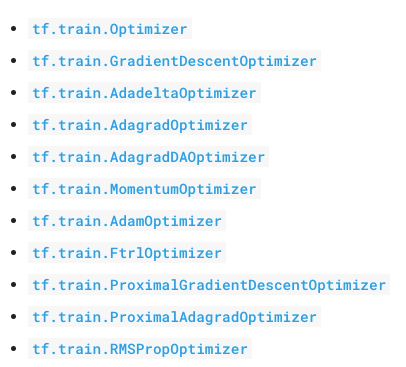W += - α * dx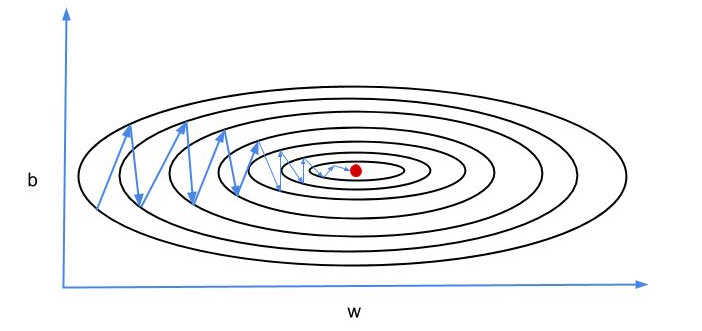#### 2. Momentum

m = b1*m - α * dx
W += m

v = dx^2
W += -(α/sqrt(v)) * dx

#### 4. RMSProp

v = b1 * v + (1-b1)*dx^2
W += -(α/sqrt(v)) * dx

m = b1m + (1-b1)dx
v = b2v + (1-b2)dx^2
W += -(α*m/sqrt(v)) * dx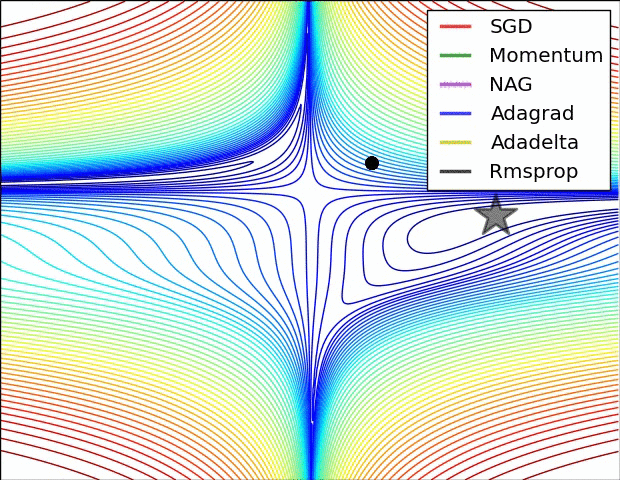《TensorFlow技术解析与实战》学习笔记2
《TensorFlow技术解析与实战》学习笔记2
32 0《TensorFlow深度学习应用实践》学习笔记1
《TensorFlow深度学习应用实践》学习笔记1
34 0《TensorFlow技术解析与实战》学习笔记1
《TensorFlow技术解析与实战》学习笔记1
23 0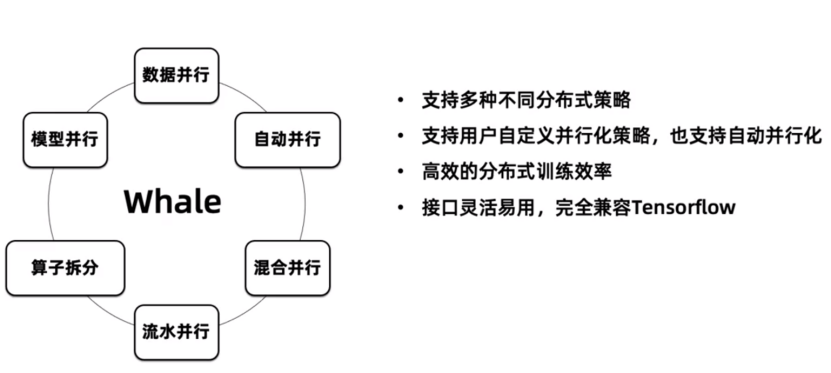Whale 基于 Tensorflow 深度学习分布式训练框架|学习笔记

108 0Tensorflow 课程框架介绍 | 学习笔记

23 0Tensorflow 课程简介 | 学习笔记

29 0167951711524

AI语音技能云开发实践-1024程序员节创造营公益课15552884961

【科技少年】AI领航员探索教程（赛前训练）302455